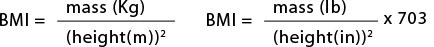###The body mass index (BMI) is defined as the individual's body mass divided by the square of his or her height. BMI can also be determined using a BMI chart, which displays BMI as a function of weight (horizontal axis) and height (vertical axis) using contour lines for different values of BMI or colors for different BMI categories.The factor for UK/US units is more precisely 703.06957964, but it is not logical or relevant to calculate a result to more precision than the input data. To work from stone and pounds first multiply the stone by 14 then add the pounds to give the whole mass in pounds; to work from feet and inches first multiply the feet by 12 then add the inches to give the whole height in inches.